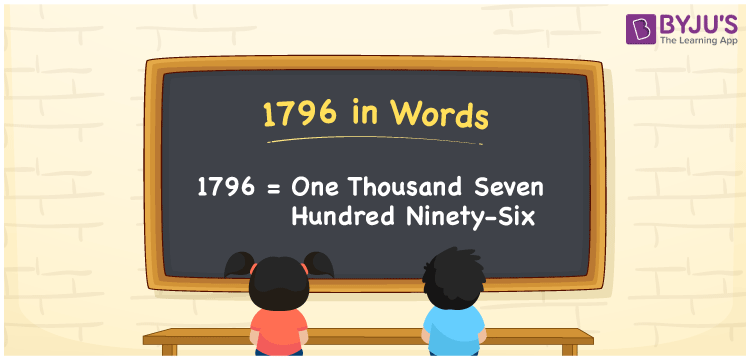# 1796 in Words

The number 1796 is written as One Thousand Seven Hundred Ninety-Six. For example, Juhi spent Rs. 1796 for a meal at a restaurant, then you can say, “Juhi spent Rupees One Thousand Seven Hundred Ninety-Six for a meal at a restaurant”. We can convert the cardinal number 1796 easily into word form with the help of a place value chart. Let us understand the procedure of converting 1796 into words using simple tricks in this article.

 1796 in Words One Thousand Seven Hundred Ninety-Six One Thousand Seven Hundred Ninety-Six in numerical form 1796

## 1796 in English Words

We generally write numbers in words using the letters of the English alphabet. Hence, the number 1796 in English is written as One Thousand Seven Hundred Ninety-Six.## How to Write 1796 in Words?

The number 1796 is a four-digit number. Hence, we need a place value table of 4 columns as shown below.

 Thousands Hundreds Tens Ones 1 7 9 6

Therefore, we can write the expanded form as:

1 x Thousand + 7 x Hundred + 9 x Ten + 6 x One

= 1 x 1000 + 7 x 100 + 9 x 10 + 6 x 1

= 1000 + 700 + 90 + 6

= 1796

= One Thousand Seven Hundred Ninety-Six

Hence, 1796 in words is written as One Thousand Seven Hundred Ninety-Six

Interesting way of writing 1796 in words

1 = One

17 = Seventeen

179 = One Hundred and Seventy-Nine

1796 = One Thousand Seven Hundred Ninety-Six

Thus, the word form of the number 1796 is One Thousand Seven Hundred Ninety-Six

1796 is a natural number that precedes 1797 and succeeds 1795

• 1796 in words – One Thousand Seven Hundred Ninety-Six
• Is 1796 an odd number? – No
• Is 1796 an even number? – Yes
• Is 1796 a perfect square number? – No
• Is 1796 a perfect cube number? – No
• Is 1796 a prime number? – No
• Is 1796 a composite number? – Yes

## Frequently Asked Questions on 1796 in Words

Q1

### How to write 1796 in words?

1796 in words is written as One Thousand Seven Hundred Ninety-Six.
Q2

### Simplify 1000 + 796, and express in words.

Simplifying 1000 + 796, we get 1796. Therefore, 1796 in words is One Thousand Seven Hundred Ninety-Six.
Q3

### Write One Thousand Seven Hundred Ninety-Six in numbers.

One Thousand Seven Hundred Ninety-Six in numbers is 1796.